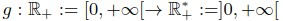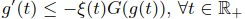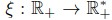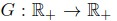## Abstract

We consider in this paper the problem of asymptotic behavior of solutions for two viscoelastic wave equations with infinite memory. We show that the stability of the system holds for a much larger class of kernels and get better decay rate than the ones known in the literature. More precisely, we consider infinite memory kernelssatisfying, whereandare given functions. Under this very general assumption on the behavior of g at infinity and for each viscoelastic wave equation, we provide a relation between the decay rate of the solutions and the growth of g at infinity, which improves the decay rates obtained in [15, 16, 17, 19, 40]. Moreover, we drop the boundedness assumptions on the history data considered in [15, 16, 17, 40].

How to Cite
Guesmia, A. (2020). New general decay rates of solutions for two viscoelastic wave equations with infinite memory. Mathematical Modelling and Analysis, 25(3), 351-373. https://doi.org/10.3846/mma.2020.10458
Published in Issue
May 13, 2020
Abstract Views
1257
807

## References

J.A. Appleby, M. Fabrizio, B. Lazzari and D.W. Reynolds. On exponential asymptotic stability in linear viscoelasticity. Mathematical Models and Methods in Applied Sciences, 16(10):1677–1694, 2006. https://doi.org/10.1142/S0218202506001674

V.I. Arnold. Mathematical methods of classical mechanics. Springer Science & Business Media, 2013.

S. Berrimi and S.A. Messaoudi. Exponential decay of solutions to a viscoelastic equation with nonlinear localized damping. Electronic Journal of Differential Equations, 88:1–10, 2004.

M. Cavalcanti, V.N. Domingos Cavalcanti and J. Ferreira. Existence and uniform decay for a non-linear viscoelastic equation with strong damping. Mathematical Methods in the Applied Sciences, 24(14):1043–1053, 2001. https://doi.org/10.1002/mma.250

M. Cavalcanti and A. Guesmia. General decay rates of solutions to a nonlinear wave equation with boundary condition of memory type. Differential and Integral Equations, 18:583–600, 2005.

M.M. Cavalcanti, V.N. Domingos Cavalcanti and J.A. Soriano. Exponential decay for the solution of semilinear viscoelastic wave equations with localized damping. Electronic Journal of Differential Equations, 44:1–14, 2002.

M.M. Cavalcanti and H.P. Oquendo. Frictional versus viscoelastic damping in a semilinear wave equation. SIAM Journal on Control and Optimization, 42(4):1310–1324, 2003. https://doi.org/10.1137/S0363012902408010

R. Christensen. Theory of viscoelasticity: an introduction. Elsevier, 2012.

M. Conti and V. Pata. Weakly dissipative semilinear equations of viscoelasticity. Communications on Pure and Applied Analysis, 4(4):705–720, 2005. https://doi.org/10.3934/cpaa.2005.4.705

C.M. Dafermos. An abstract Volterra equation with applications to linear viscoelasticity. Journal of Differential Equations, 7(3):554–569, 1970. https://doi.org/10.1016/0022-0396(70)90101-4

C.M. Dafermos. Asymptotic stability in viscoelasticity. Archive for Rational Mechanics and Analysis, 37:297–308, 1970. https://doi.org/10.1007/BF00251609

G. Dassios and F. Zafiropoulos. Equipartition of energy in linearized 3d viscoelasticity. Quarterly of Applied Mathematics, 48:715–730, 1990. https://doi.org/10.1090/qam/1079915

C. Giorgi, J.E. Muñoz Rivera and V. Pata. Global attractors for a semilinear hyperbolic equation in viscoelasticity. Journal of Mathematical Analysis and Applications, 260(1):83–99, 2001. https://doi.org/10.1006/jmaa.2001.7437

A. Guesmia. Stabilisation de l’équation des ondes avec conditions aux limites de type m´emoire. Afrika Mathematika, 10:14–25, 1999.

A. Guesmia. Asymptotic stability of abstract dissipative systems with infinite memory. Journal of Mathematical Analysis and Applications, 382(2):748–760, 2011. https://doi.org/10.1016/j.jmaa.2011.04.079

A. Guesmia. Asymptotic behavior for coupled abstract evolution equations with one infinite memory. Applicable Analysis, 94:184–217, 2015. https://doi.org/10.1080/00036811.2014.890708

A. Guesmia and S. Messaoudi. A new approach to the stability of an abstract system in the presence of infinite history. Journal of Mathematical Analysis and Applications, 416:212–228, 2014. https://doi.org/10.1016/j.jmaa.2014.02.030

A. Guesmia and S.A. Messaoudi. A general decay result for a viscoelastic equation in the presence of past and finite history memories. Nonlinear Analysis: Real World Applications, 13(1):476–485, 2012. https://doi.org/10.1016/j.nonrwa.2011.08.004

A. Guesmia and N. Tatar. Some well-posedness and stability results for abstract hyperbolic equations with infinite memory and distributed time delay. Communications on Pure and Applied Analysis, 14(2):457–491, 2015. https://doi.org/10.3934/cpaa.2015.14.457

J.H. Hao and F. Wang. General decay rate for weak viscoelastic wave equation with Balakrishnan-Taylor damping and time-varying delay. Computers and Mathematics with Applications, 78(9):2632–2640, 2019. https://doi.org/10.1016/j.camwa.2019.04.010

J.H. Hao and P. Wang. General stability result of abstract thermoelastic system with infinite memory. Bulletin of the Malaysian Mathematical Sciences Society, 42:2549–2567, 2019. https://doi.org/10.1007/s40840-018-0615-z

J.H. Hao and J. Wei. Intrinsic decay rate estimates for abstract wave equation with memory. Mathematical Methods in the Applied Sciences, 40:5131–5140, 2017. https://doi.org/10.1002/mma.4375

J. Hashim Hassan and S.A. Messaoudi. General decay rate for a class of weakly dissipative second-order systems with memory. Mathematical Methods in the Applied Sciences, 42:2842–2853, 2019. https://doi.org/10.1002/mma.5554

W.J. Hrusa. Global existence and asymptotic stability for a semilinear hyperbolic Volterra equation with large initial data. SIAM Journal on Mathematical Analysis, 16(1):110–134, 1985. https://doi.org//10.1137/0516007

K.P. Jin, J. Liang and T.J. Xiao. Coupled second order evolution equations with fading memory: optimal energy decay rate. Journal of Differential Equations, 257(5):1501–1528, 2014. https://doi.org//10.1016/j.jde.2014.05.018

K.P. Jin, J. Liang and T.J. Xiao. Asymptotic behavior of coupled systems of second order abstract evolution equations with one infinite memory. Journal of Mathematical Analysis and Applications, 475(1):554–575, 2019. https://doi.org/10.1016/j.jmaa.2019.02.055

F. Li, Z. Zhao and Y. Chen. Global existence uniqueness and decay estimates for nonlinear viscoelastic wave equation with boundary dissipation. Nonlinear Analysis: Real World Applications, 12(3):1759–1773, 2011. https://doi.org/10.1016/j.nonrwa.2010.11.009

S.A. Messaoudi. General decay of solutions of a viscoelastic equation. Journal of Mathematical Analysis and Applications, 341(2):1457–1467, 2008. https://doi.org/10.1016/j.jmaa.2007.11.048

S.A. Messaoudi. General decay of the solution energy in a viscoelastic equation with a nonlinear source. Nonlinear Analysis: Theory, Methods and Applications, 69:2589–2598, 2008. https://doi.org/10.1016/j.na.2007.08.035

S.A. Messaoudi and W. Al-Khulaifi. General and optimal decay for a quasilinear viscoelastic equation. Applied Mathematics Letters, 66:16–22, 2017. https://doi.org/10.1016/j.aml.2016.11.002

S.A. Messaoudi and N. Tatar. Global existence and asymptotic behavior for nonlinear viscoelastic problem. Mathematical Sciences Research Journal, 7:136– 149, 2003. https://doi.org/10.1002/mma.804

S.A. Messaoudi and N. Tatar. Global existence and uniform stability of solutions for a quasilinear viscoelastic problem. Mathematical Methods in the Applied Sciences, 30:665–680, 2007.

M.I. Mustafa. Optimal decay rates for the viscoelastic wave equation. Mathematical Methods in the Applied Sciences, 41(1):192–204, 2018. https://doi.org/10.1002/mma.4604

M.I. Mustafa and S.A. Messaoudi. General stability result for viscoelastic wave equations. Journal of Mathematics and Physics, 53(5), 2012. https://doi.org/10.1063/1.4711830

V. Pata. Stability and exponential stability in linear viscoelasticity. Milan Journal of Mathematics, 77:333–360, 2009. https://doi.org/10.1007/s00032-009-0098-3

A. Pazy. Semigroups of linear operators and applications to partial differential equations. Springer-Verlaag, 1983. https://doi.org/10.1007/978-1-4612-5561-1

J.E. Muñoz Revira and M. Grazia Naso. Asymptotic stability of semigroups associated with linear weak dissipative systems with memory. Journal of Mathematical Analysis and Applications, 326(1):691–707, 2007. https://doi.org/10.1016/j.jmaa.2006.03.022

J.E. Muñoz Rivera. Asymptotic behaviour in linear viscoelasticity. Quarterly of Applied Mathematics, 52:628–648, 1994. https://doi.org/10.1090/qam/1306041

B. Said-Houari, S.A. Messaoudi and A. Guesmia. General decay of solutions of a nonlinear system of viscoelastic wave equations. Nonlinear Differential Equations and Applications (NoDEA), 18:659–684, 2011. https://doi.org/10.1007/s00030-011-0112-7

A. Youkana. Stability of an abstract system with infinite history. arXiv preprint arXiv, 2018.